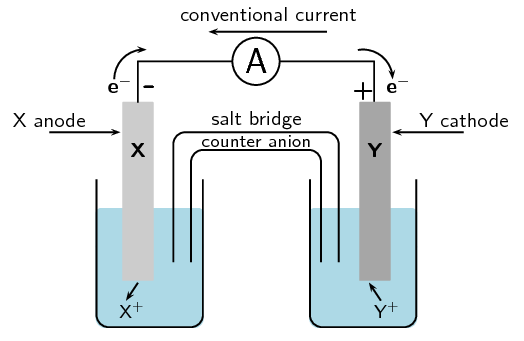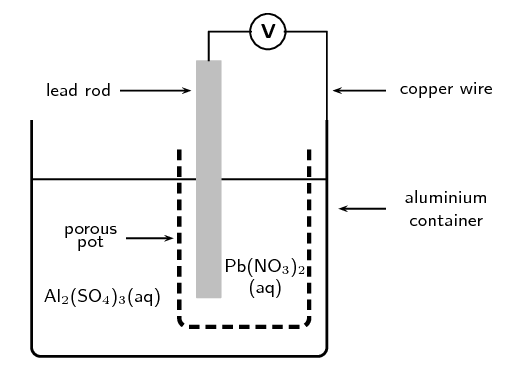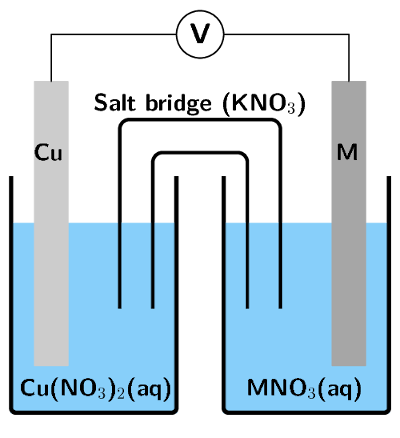Home Practice
For learners and parents For teachers and schools
Textbooks
Full catalogue
Pricing SupportLog in

We think you are located in United States. Is this correct?

# 13.8 Chapter summary

## 13.8 Chapter summary (ESCRT)

• Oxidation is the loss of electrons and reduction is the gain of electrons.

• A redox reaction is one where there is always a change in the oxidation numbers of the elements that are involved in the reaction.

• It is possible to balance redox equations using the half-reactions that take place within the overall reaction.

• An electrochemical reaction is one where either a chemical reaction produces an electric current, or where an electric current causes a chemical reaction to take place.

• In a galvanic cell a chemical reaction produces a current in the external circuit. An example is the zinc-copper cell.

• An electrolytic cell is an electrochemical cell that uses electricity to drive a non-spontaneous reaction. In an electrolytic cell, electrolysis occurs, which is a process of separating elements and compounds using an electric current.

• Cells have a number of components. They consist of two electrodes, which are connected to each other by an external circuit wire.

• In a galvanic cell each electrode is placed in a separate container in an electrolyte solution. The two electrolytes are connected by a salt bridge.

• In an electrolytic cell both electrodes are placed in the same container in an electrolyte solution.

• One of the electrodes is the anode, where oxidation takes place. The cathode is the electrode where reduction takes place.

• In a galvanic cell, the build up of electrons at the anode sets up a potential difference between the two electrodes, and this causes a current to flow in the external circuit.

• Standard cell notation for a galvanic cell has the anode on the left and the cathode on the right. For example:

$$\text{Zn}(\text{s})|\text{Zn}^{2+}(\text{aq})||\text{Cu}^{2+}(\text{aq})|\text{Cu}(\text{s})$$

$$|$$ = a phase boundary (solid/aqueous)

$$||$$ = the salt bridge

• Different metals have different reaction potentials. The reduction potential of metals (in other words, their ability to ionise), is recorded in a table of standard electrode reduction potentials. The more negative the value, the greater the tendency of the metal to be oxidised. The more positive the value, the greater the tendency of the metal to be reduced.

• The values on the table of standard electrode potentials are measured relative to the standard hydrogen electrode.

• The EMF of a cell can be calculated using one of the following equations:

E°(cell) = E°(reduction half-reaction) - E°(oxidation half-reaction)

E°(cell) = E°(oxidising agent) - E°(reducing agent)

E°(cell) = E°(cathode) - E°(anode)

• It is possible to predict whether a reaction is spontaneous or not, either by looking at the sign of the cell EMF or by comparing the electrode potentials of the two half-cells.

• Industrial applications of cells include electrolysis (the electrowinning of copper), in the chloralkali industry (mercury, diaphragm and membrane cells), as well as the extraction of metals from ores (e.g. aluminium from bauxite).

A comparison of galvanic and electrolytic cells

It should be much clearer now that there are a number of differences between galvanic and electrolytic cells. Some of these differences have been summarised in Table 13.5.

 Galvanic cell Electrolytic cell Chemical reactions spontaneous reactions non-spontaneous reactions Energy changes Chemical potential energy from chemical reactions is converted to electrical energy An external supply of electrical energy causes a chemical reaction to occur Anode is negative, oxidation occurs at anode is positive, oxidation occurs at anode Cathode is positive, reduction occurs at cathode is negative, reduction occurs at cathode Cell set-up two half-cells, one electrode in each, connected by a salt-bridge one cell, both electrodes in cell, no salt-bridge Electrolyte solution(s) The electrolyte solutions are kept separate from one another, and are connected by a salt bridge The cathode and anode are in the same electrolyte Applications batteries Electrolysis e.g. of water, NaCl, electroplating

Table 13.5: A comparison of galvanic and electrolytic cells.

temp text
Textbook Exercise 13.11

A cell is set up. There is a negative charge on the anode.

What type of cell is this?

Galvanic cell

What type of reaction is occurring at the cathode?

Reduction

Can the electrodes have the same reaction potential?

No, a galvanic cell requires two electrodes with different reaction potentials (different metals).

Draw and label a diagram of this cell.For each of the following, say whether the statement is true or false. If it is false, re-write the statement correctly.

The anode in an electrolytic cell has a negative charge.

False. The anode in an electrolytic cell has a positive charge.

The reaction $$2\text{KClO}_{3}(\text{s})$$ $$\to$$ $$2\text{KCl}(\text{s}) + 3\text{O}_{2}(\text{g})$$ is an example of a redox reaction.

True

Lead is a stronger oxidising agent than nickel.

True

Sulfur dioxide gas can be prepared in the laboratory by heating a mixture of copper turnings and concentrated sulfuric acid in a suitable flask.

Derive a balanced ionic equation for this reaction using the half-reactions that take place.

The reaction uses copper and concentrated sulfuric acid and produces sulfur dioxide. Look for a suitable reaction on the table of standard electrode potentials:

$$\text{SO}_{4}^{2-}(\text{aq}) + 4\text{H}^{+}(\text{aq}) + 2\text{e}^{-}$$ $$\rightleftharpoons$$ $$\text{SO}_{2}(\text{g}) + 2\text{H}_{2}\text{O}(\text{ℓ})$$ (E$$^{\circ}$$ = $$\text{+0,17}$$ $$\text{V}$$)

$$\text{Cu}^{2+}(\text{aq}) + 2\text{e}^{-}$$ $$\rightleftharpoons$$ $$\text{Cu}(\text{s})$$ (E$$^{\circ}$$ = $$\text{+0,34}$$ $$\text{V}$$)

The reactants must be copper and concentrated sulfuric acid, therefore:

Reduction half-reaction: $$\text{SO}_{4}^{2-}(\text{aq}) + 4\text{H}^{+}(\text{aq}) + 2\text{e}^{-}$$ $$\to$$ $$\text{SO}_{2}(\text{g}) + 2\text{H}_{2}\text{O}(\text{ℓ})$$

Oxidation half-reaction: $$\text{Cu}(\text{s})$$ $$\to$$ $$\text{Cu}^{2+}(\text{aq}) + 2\text{e}^{-}$$

Adding the two half-reactions together and cancelling out the electrons (the charge balances) gives us:

$$\text{SO}_{4}^{2-}(\text{aq}) + 4\text{H}^{+}(\text{aq}) + \text{Cu}(\text{s})$$ $$\to$$ $$\text{SO}_{2}(\text{g}) + 2\text{H}_{2}\text{O}(\text{ℓ}) + \text{Cu}^{2+}(\text{aq})$$

Give the E° value for the overall reaction.

E°$$_\text{(cell)}$$ = E°$$_\text{(reduction)}$$ - E°$$_\text{(oxidation)}$$ = $$\text{0,17}$$ - $$\text{0,34}$$ = $$-\text{0,17}$$ $$\text{V}$$

Explain why it is necessary to heat the reaction mixture.

The EMF of the cell is negative, so the reaction is non-spontaneous. Therefore, heat will be needed to make the reaction happen.

(IEB Paper 2, 2002)

For each of the following questions, choose the one correct answer.

Which one of the following reactions is a redox reaction?

1. $$\text{HCl}(\text{aq}) + \text{NaOH}(\text{aq})$$ $$\to$$ $$\text{NaCl}(\text{aq}) + \text{H}_{2}\text{O}(\text{ℓ})$$

2. $$\text{AgNO}_{3}(\text{s}) + \text{NaI}(\text{s})$$ $$\to$$ $$\text{AgI}(\text{s}) + \text{NaNO}_{3}(\text{s})$$

3. $$2\text{FeCl}_{3}(\text{aq}) + 2\text{H}_{2}\text{O}(\text{ℓ}) + \text{SO}_{2}(\text{aq})$$ $$\to$$ $$\text{H}_{2}\text{SO}_{4}(\text{aq}) + 2\text{HCl}(\text{aq}) + 2\text{FeCl}_{2}(\text{aq})$$

4. $$\text{BaCl}_{2}(\text{s}) + \text{MgSO}_{4}(\text{s})$$ $$\to$$ $$\text{MgCl}_{2}(\text{s}) + \text{BaSO}_{4}(\text{s})$$

(IEB Paper 2, 2003)

Only (iii) has both oxidation and reduction occurring (i.e. a change in the oxidation numbers).

iii) $$2\text{FeCl}_{3}(\text{s}) + 2\text{H}_{2}\text{O}(\text{ℓ}) + \text{SO}_{2}(\text{aq})$$ $$\to$$ $$\text{H}_{2}\text{SO}_{4}(\text{aq}) + 2\text{HCl} + 2\text{FeCl}_{2}(\text{aq})$$

Consider the reaction represented by the following equation:

$$\text{Br}_{2}(\text{ℓ}) + 2\text{I}^{-}(\text{aq})$$ $$\to$$ $$2\text{Br}^{-}(\text{aq}) + \text{I}_{2}(\text{s})$$

1. bromine is oxidised

2. bromine acts as a reducing agent

3. the iodide ions are oxidised

4. iodine acts as a reducing agent

(IEB Paper 2, 2002)

Bromine gains electrons (is reduced), therefore bromine acts as a oxidising agent. Iodide loses electrons (is oxidised), therefore iodide acts as a reducing agent. In the reverse reaction iodine would gain electrons (be reduced) and act as an oxidising agent.

iii) the iodide ions are oxidised

The following equations represent two hypothetical half-reactions:

$$\text{X}_{2} + 2\text{e}^{-}$$ $$\rightleftharpoons$$ $$2\text{X}^{-}$$ (E$$^{\circ}$$ = $$\text{+1,09}$$ $$\text{V}$$)

and

$$\text{Y}^{+} + \text{e}^{-}$$ $$\rightleftharpoons$$ $$\text{Y}$$ (E$$^{\circ}$$ = $$-\text{2,80}$$ $$\text{V}$$)

Which one of the following substances from these half-reactions has the greatest tendency to lose electrons?

1. $$\text{X}^{-}$$

2. $$\text{X}_{2}$$

3. $$\text{Y}$$

4. $$\text{Y}^{+}$$

Loss of electrons is oxidation. The larger, negative value will be oxidised more easily. $$\text{Y}$$ $$\to$$ $$\text{Y}^{+} + \text{e}^{-}$$

iii) $$\text{Y}$$

Which one of the following redox reactions will not occur spontaneously at room temperature?

1. $$\text{Mn}(\text{s}) + \text{Cu}^{2+}(\text{aq})$$ $$\to$$ $$\text{Mn}^{2+}(\text{aq}) + \text{Cu}(\text{s})$$

2. $$\text{Fe}^{3+}(\text{aq}) + 3\text{NO}_{2}(\text{g}) + 3\text{H}_{2}\text{O}(\text{ℓ})$$ $$\to$$ $$\text{Fe}(\text{s}) + 3\text{NO}_{3}^{-}(\text{aq}) + 6\text{H}^{+}(\text{aq})$$

3. $$\text{Zn}(\text{s}) + \text{SO}_{4}^{2-}(\text{aq}) + 4\text{H}^{+}(\text{aq})$$ $$\to$$ $$\text{Zn}^{2+}(\text{aq}) + \text{SO}_{2}(\text{g}) + 2\text{H}_{2}\text{O}(\text{ℓ})$$

4. $$5\text{H}_{2}\text{S}(\text{g}) + 2\text{MnO}_{4}^{-}(\text{aq}) + 6\text{H}^{+}(\text{aq})$$ $$\to$$ $$5\text{S}(\text{s}) + 2\text{Mn}^{2+}(\text{aq}) + 8\text{H}_{2}\text{O}(\text{ℓ})$$

(DoE Exemplar Paper 2, 2008)

E°$$_\text{(cell)}$$ = E°$$_\text{(reduction)}$$ - E°$$_\text{(oxidation)}$$

E°$$_\text{(cell)}$$ for (i) = E°$$_\text{(copper)}$$ - E°$$_\text{(manganese)}$$ = $$\text{0,34} - (-\text{1,18}$$) = $$\text{+1,52}$$ $$\text{V}$$.

Therefore spontaneous.

E°$$_\text{(cell)}$$ for (ii) = E°$$_\text{(iron)}$$ - E° $$_\text{(nitrogen dioxide)}$$ = ($$-\text{0,06}$$) - $$\text{0,80}$$ = $$-\text{0,86}$$ $$\text{V}$$.

Therefore non-spontaneous.

E°$$_\text{(cell)}$$ for (iii) = E°$$_\text{(sulfate ion)}$$ - E°$$_\text{(zinc)}$$ = $$\text{0,17} - (-\text{0,76}$$) = $$\text{+0,93}$$ $$\text{V}$$.

Therefore spontaneous.

E°$$_\text{(cell)}$$ for (iv) = E°$$_\text{(permanganate ion)}$$ - E°$$_\text{(hydrogen sulfide)}$$ = $$\text{1,51} - \text{0,14}$$ = $$\text{+1,37}$$ $$\text{V}$$.

Therefore spontaneous.

Correct: ii) $$\text{Fe}^{3+}(\text{aq}) + 3\text{NO}_{2}(\text{g}) + 3\text{H}_{2}\text{O}(\text{ℓ})$$ $$\to$$ $$\text{Fe}(\text{s}) + 3\text{NO}_{3}^{-}(\text{aq}) + 6\text{H}^{+}(\text{aq})$$

In order to investigate the rate at which a reaction proceeds, a learner places a beaker containing concentrated nitric acid on a sensitive balance. A few pieces of copper metal are dropped into the nitric acid.

Use the relevant half-reactions from the table of standard electrode potentials to derive the balanced net ionic equation for the reaction that takes place in the beaker.

The reaction has nitric acid as a reactant, nitrogen monoxide will be a product. Find a suitable reaction on the table of standard electrode potentials:

Reduction half-reaction: $$\text{NO}_{3}^{-}(\text{aq}) + 4\text{H}^{+}(\text{aq}) + 3\text{e}^{-}$$ $$\to$$ $$\text{NO}(\text{g}) + 2\text{H}_{2}\text{O}(\text{ℓ})$$

The reaction has solid copper as a reactant, so this must be the oxidation half-reaction:

Oxidation half-reaction: $$\text{Cu}(\text{s})$$ $$\to$$ $$\text{Cu}^{2+}(\text{aq}) + 2\text{e}^{-}$$

We need to multipy the reduction half-reaction by 2 and the oxidation half-reaction by 3 to balance the charge:

$$2\text{NO}_{3}^{-}(\text{aq}) + 8\text{H}^{+}(\text{aq}) + 6\text{e}^{-}$$ $$\to$$ $$2\text{NO}(\text{g}) + 4\text{H}_{2}\text{O}(\text{ℓ})$$

$$3\text{Cu}(\text{s})$$ $$\to$$ $$3\text{Cu}^{2+}(\text{aq}) + 6\text{e}^{-}$$

to get:

$$2\text{NO}_{3}^{-}(\text{aq}) + 8\text{H}^{+}(\text{aq}) + 3\text{Cu}(\text{s})$$ $$\to$$ $$2\text{NO}(\text{g}) + 4\text{H}_{2}\text{O}(\text{ℓ}) + 3\text{Cu}^{2+}(\text{aq})$$

What chemical property of nitric acid is illustrated by this reaction?

Nitric acid is being reduced, and can act as an oxidising agent.

List three observations that this learner would make during the investigation.

(IEB Paper 2, 2005)

The learner would observe:

• a (brown) gas bubbling in the solution

• a colour change (the solution changing from colourless to green)

• a mass change

The following reaction takes place in an electrochemical cell:

$$\text{Cu}(\text{s}) + 2\text{AgNO}_{3}(\text{aq})$$ $$\to$$ $$\text{Cu}(\text{NO}_{3})_{2}(\text{aq}) + 2\text{Ag}(\text{s})$$

Give an equation for the oxidation half-reaction.

$$\text{Cu}(\text{s})$$ $$\to$$ $$\text{Cu}^{2+}(\text{aq}) + 2\text{e}^{-}$$

Which metal is used as the anode?

Oxidation is loss at the anode. Therefore copper is the anode.

Determine the EMF of the cell under standard conditions.

(IEB Paper 2, 2003)

$$\text{Cu}^{2+}(\text{aq}) + 2\text{e}^{-}$$ $$\rightleftharpoons$$ $$\text{Cu}(\text{s})$$ (E$$^{\circ}$$ = $$\text{+0,34}$$ $$\text{V}$$)

$$\text{Ag}^{+}(\text{aq}) + \text{e}^{-}$$ $$\rightleftharpoons$$ $$\text{Ag}(\text{s})$$ (E$$^{\circ}$$ = $$\text{+0,80}$$ $$\text{V}$$)

E°$$_\text{(cell)}$$ = E°$$_\text{(reduction)}$$ - E°$$_\text{(oxidation)}$$

E°$$_\text{(cell)}$$ = E°$$_\text{(silver)}$$ - E°$$_\text{(copper)}$$ = $$\text{0,80}$$ - $$\text{0,34}$$ = $$\text{0,46}$$ $$\text{V}$$

A galvanic cell is constructed by placing a lead rod in a porous pot containing a solution of lead nitrate. The porous pot is then placed in a large aluminium container filled with a solution of aluminium sulfate. The lead rod is then connected to the aluminium container by a copper wire and voltmeter as shown.Define the term reduction.

Reduction is the gain of electrons by an element or ion.

Write balanced equations for the reactions that take place at...

the cathode

Lead has a smaller, negative E° ($$-\text{0,13}$$ $$\text{V}$$) than aluminium ($$-\text{1,66}$$ $$\text{V}$$). Therefore lead is more likely to be reduced. Reduction is gain of electrons at the cathode.

$$\text{Pb}^{2+}(\text{aq}) + 2\text{e}^{-}$$ $$\to$$ $$\text{Pb}(\text{s})$$

the anode

Aluminium has a larger, negative E° ($$-\text{1,66}$$ $$\text{V}$$) than lead ($$-\text{0,13}$$ $$\text{V}$$). Therefore aluminium is more likely to be oxidised. Oxidation is loss of electrons at the anode.

$$\text{Al}(\text{s})$$ $$\to$$ $$\text{Al}^{3+}(\text{aq}) + 3\text{e}^{-}$$

Write a balanced net ionic equation for the reaction which takes place in this cell.

Balance the charge by multiplying the reduction half-reaction by $$\text{3}$$, and the oxidation half-reaction by $$\text{2}$$:

$$3\text{Pb}^{2+}(\text{aq}) + 6\text{e}^{-}$$ $$\to$$ $$3\text{Pb}(\text{s})$$

$$2\text{Al}(\text{s})$$ $$\to$$ $$2\text{Al}^{3+}(\text{aq}) + 6\text{e}^{-}$$

Therefore the overal equation is:

$$3\text{Pb}^{2+}(\text{aq}) + 2\text{Al}(\text{s})$$ $$\to$$ $$3\text{Pb}(\text{s}) + 2\text{Al}^{3+}(\text{aq})$$

In which direction do electrons flow in the copper wire? ($$\text{Al}$$ to $$\text{Pb}$$ or $$\text{Pb}$$ to $$\text{Al}$$)

In a galvanic cell the anode is negative and the cathode is positive. Therefore electrons flow from the anode to the cathode. From $$\text{Al}$$ to $$\text{Pb}$$.

What are the two functions of the porous pot?

The porous pot allows the flow of ions while also keeping the two solutions separate.

Calculate the EMF of this cell under standard conditions.

(IEB Paper 2, 2005)

E°$$_\text{(cell)}$$ = E°$$_\text{(cathode)}$$ - E°$$_\text{(anode)}$$

E°$$_\text{(cell)}$$ = E°$$_\text{(lead)}$$ - E°$$_\text{(aluminium)}$$ = $$-\text{0,13} - (-\text{1,66})$$ = $$\text{+1,53}$$ $$\text{V}$$

An electrochemical cell is made up of a copper electrode in contact with a copper nitrate solution and an electrode made of an unknown metal $$\text{M}$$ in contact with a solution of $$\text{MNO}_{3}$$. A salt bridge containing a $$\text{KNO}_{3}$$ solution joins the two half-cells. A voltmeter is connected across the electrodes. Under standard conditions the reading on the voltmeter is $$\text{+0,46}$$ $$\text{V}$$.

Sketch the electrochemical cell.Write down the standard conditions which apply to this electrochemical cell.

Pressure = $$\text{101,3}$$ $$\text{kPa}$$

Concentration = $$\text{1}$$ $$\text{mol·dm^{-3}}$$

Temperature = $$\text{298}$$ $$\text{K}$$

$$\text{Cu}(\text{s})$$ $$\to$$ $$\text{Cu}^{2+}(\text{aq}) + 2\text{e}^{-}$$. Identify the metal $$\text{M}$$. Show calculations.

E°$$_{\text{(cell)}} =$$E°$$_\text{(reduction)}$$ - E°$$_\text{(oxidation)}$$

$$\text{Cu}^{2+}(\text{aq}) + 2\text{e}^{-}$$ $$\rightleftharpoons$$ $$\text{Cu}(\text{s})$$ (E$$^{\circ}$$ = $$\text{+0,34}$$ $$\text{V}$$)

We know the EMF of the cell (that is the reading on the voltmeter), we are also shown that the copper reaction is the oxidation half-reaction.

$$\text{+0,46}$$ = E°$$_\text{(reduction)}$$ - $$\text{0,34}$$

E°$$_\text{(reduction)}$$ = $$\text{0,46}$$ + $$\text{0,34}$$ = $$\text{+0,80}$$ $$\text{V}$$

Now we look at the table of standard potentials to find the unknown metal. The metal is silver ($$\text{Ag}$$).

Use the standard electrode potentials to write down equations for the:

anode half-reaction

Oxidation is loss (of electrons) at the anode. Therefore:

$$\text{Cu}(\text{s})$$ $$\to$$ $$\text{Cu}^{2+}(\text{aq}) + 2\text{e}^{-}$$

cathode half-reaction

Reduction is gain (of electrons) at the cathode. Therefore:

$$\text{Ag}^{+}(\text{aq}) + \text{e}^{-}$$ $$\to$$ $$\text{Ag}(\text{s})$$

overall cell reaction

To balance the charge the reduction half-reaction (silver) needs to be multiplied by $$\text{2}$$:

$$2\text{Ag}^{+}(\text{aq}) + 2\text{e}^{-}$$ $$\to$$ $$2\text{Ag}(\text{s})$$

Therefore:

$$\text{Cu}(\text{s}) + 2\text{Ag}^{+}(\text{aq})$$ $$\to$$ $$\text{Cu}^{2+}(\text{aq}) + 2\text{Ag}(\text{s})$$

What is the purpose of the salt bridge?

(IEB Paper 2, 2004)

The salt bridge acts as a transfer medium that allows ions to flow through without allowing the different solutions to mix and react. It completes the circuit and maintains the neutrality of the half-cell solutions.